Students can Download Science Chapter 1 Measurement Questions and Answers, Notes Pdf, Samacheer Kalvi 7th Science Book Solutions Guide Pdf helps you to revise the complete Tamilnadu State Board New Syllabus and score more marks in your examinations.

## Tamilnadu Samacheer Kalvi 7th Science Solutions Term 1 Chapter 1 Measurement

### Samacheer Kalvi 7th Science Measurement Textual Evaluation

Samacheer Kalvi Guru 7th Science Question 1.
Which of the following is a derived unit?
(a) mass
(b) time
(c) area
(d) length
(c) area

Measurement 7th Standard Science Question 2.
Which of the following is correct?
(a) 1L=lcc
(b) 1L= l0cc
(c) 1L= l00cc
(d) 1L= l000cc
(d) 1L = 1000cc

Measurement Lesson 7th Standard Question 3.
SI unit of density is
(a) kg/m2
(b) kg/m3
(c) kg/m
(d) g/m3
(b) kg/m3

7th Science Measurement Question 4.
Two spheres have equal mass and volume in the ratio 2:1. The ratio of their density is
(a) 1:2
(b) 2:1
(c) 4:1
(d) 1:4
(b) 2:1

Samacheer Kalvi 7th Science Solutions Term 1 Chapter 1 Measurement Question 5.
Light year is the unit of
(a) Distance
(b) time
(c) density
(d) both length and time
(a) Distance

II. Fill in the blanks:

1. Volume of irregularly shaped objects are measured using the law of ___________
2. One cubic metre is equal to ___________ cubic centimetre.
3. Density of mercury is ___________
4. One astronomical unit is equal to ___________
5. The area of a leaf can be measured using a ___________

1. Archimedes
2. 10,00,000 or 1066
3. 13,600 kg/m3
4. 1.496×1011m
5. graph sheet

III. State whether the following statements are true or false.

7th Standard Science Measurement Question 1.
The region covered by the boundary of the plane figure is called its volume.
(False) Correct statement: The region covered by the boundary of plane figure is called its area.

7th Standard Measurement Question 2.
Volume of liquids can be found using measuring containers.
True

Science Measurement Lesson Question Answer Question 3.
Water is denser than kerosene.
True

Unit 1 Measurement Question 4.
A ball of iron floats in mercury.
True

Measurement Lesson Question Answer Question 5.
A substance which contains less number of molecules per unit volume is said to be denser.
False. Correct statement: A substance which contains more number of molecules per unit volume is said to be denser.

IV. Match the items in column – I to the items in column – II :

Samacheer Guru 7th Science Question 1.

 Column -1 Column – II i. Area (a) light year ii. Distance (b) m3 iii. Density (c) m2 iv. Volume (d) kg V. Mass (e) kg/ m3

1. i
2. ii
3. iii
4. iv
5. v

7th Standard Measurement Lesson Question 2.

 Column -1 Column – II i. Area (a) g / cm3 ii. Length (b) measuring jar iii. Density (c) amount of a substance iv. Volume (d) rope V. Mass (e) plane figures

1. i
2. ii
3. iii
4. iv
5. v

V. Arrange the following in correct sequence :

Samacheer Kalvi 7th Science Guide Question 1.
1L, 100 cc, 10 L, 10 cc
10 cc, 100 cc, 1L, 10L

Samacheer Kalvi 7th Science Question 2.
Copper, Aluminium, Gold, Iron
Aluminium, Iron, Copper, Gold

VI. Use the analogy to fill in the blank:

Samacheer Kalvi 7th Science Solution Question 1.
Area: M2 :: Volume : _________
M3

Samacheer Kalvi 7th Science Answers Question 2.
Liquid : Litre :: Solid : _________
cm3

7th Standard Science Unit 1 Measurement Question 3.
Water: Kerosene :: ______ : Aluminium
Iron

VII. Assertion and reason type questions:
Mark the correct choice as

(a) Both assertion and reason are true and reason is the correct explanation of assertion
(b) Both assertion and reason are true, but reason is not the correct explanation of assertion
(c) If assertion is true but reason is false
(d) Assertion is false but reason is true.

7th Measurements Question 1.
Assertion (A) : Volume of a stone is found using a measuring cylinder.
Reason (R) : Stone is an irregularly shaped object.
(a) If both assertion and reason are true and reason is the correct explanation of assertion

Class 7 Science Measurement Question 2.
Assertion (A) : Wood floats in water.
Reason (R) : Water is a transparent liquid.
(b) If both assertion and reason are true, but reason is not the correct explanation of assertion
Correct explanation: Density of water is more than the density of wood.

Samacheer Kalvi.Guru 7th Science Question 3.
Assertion (A) : Iron ball sinks in water.
Reason (R) : Water is denser than iron.
(b) If both assertion and reason are true, but reason is not the correct explanation of assertion
Correct explanation : Density of iron is more than that of water.

Samacheer Kalvi Guru 7th Science Book Back Answers Question 1.
Name some of the derived quantities.
Area, volume, density.

Samacheer Kalvi Guru 7th Standard Science Question 2.
Give the value of one light year.
One light year = 9.46 x 1015m

Samacheer Kalvi Guru Science 7th Question 3.
Write down the formula used to find the volume of a cylinder.
Volume of a cylinder = πr2 h

Question 4.
Give the formula to find the density of objects.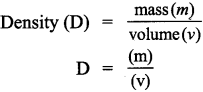Question 5.
Name the liquid in which an iron ball sinks.
Iron ball sinks in water. The density of an iron ball is more than that of water so it sinks in water.

Question 6.
Name the unit used to measure the distance between celestial objects.
Astronomical unit and light year are the units used to measure the distance between celestial objects.

Question 7.
What is the density of gold?
Density of gold is 19,300 kg/m3

Question 1.
What are derived quantities?
The physical quantities which can be obtained by multiplying, dividing or by mathematically combining the fundamental quantities are known as derived quantities.
(or)
The physical quantities which are expressed is terms of fundamental quantities are called

Question 2.
Distinguish between the volume of liquid and capacity of a container.

 S.No Volume of liquid Capacity of a container 1. Volume is the amount of space taken up by a liquid Capacity is the measure of an objects ability to hold a substance like solid, liquid or gas 2. It is measured in cubic units. It is measured in litres, gallons, pounds, etc. 3. It is calculated by multiplying the length, width and height of an object. It’s measurement is cc or ml.

Question 3.
Define the density of objects.
Density of a substance is defined as the mass of the substance contained in unit volume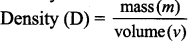Question 4.
What is one light year?
One light year is the distance travelled by light in vacuum during the period of one year.
1 Light year = 9.46 x 1015m.

Question 5.
Define one astronomical unit?
One astronomical unit is defined as the average distance between the earth and the sun.
1 AU = 1.496 5 106km = 1.496 × 1011m.

Question 1.
Describe the graphical method to find the area of an irregularly shaped plane figure.
To find the area of an irregularly shaped plane figure, we have to use graph paper.

1. Place a piece of paper with an irregular shape on a graph paper and draw its outline.
2. To find the area enclosed by the outline, count the number of squares inside it (M).
3. You will find that some squares lie partially inside the outline.
4. Count a square only if half (p) or more of it (N) lies inside the outline.
5. Finally count the number of squares, that are less than half. Let it be

For the shape in figure we have the following:
M = 50
N = 7
P = 4
Q = 4
Now, the approximate area of the can be calculated using the following formula.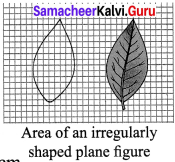Area of the leaf = M+($$\frac { 3 }{ 4 }$$) N + ($$\frac { 1 }{ 2 }$$) P+$$\frac { 1 }{ 4 }$$ Qsq.cm
= 52 + 5.25 = 58.25 sq.mm = 0.5825 sq.cm

Question 2.
How will you determine the density of a stone using a measuring jar?
Determination of density of a stone using a measuring cylinder.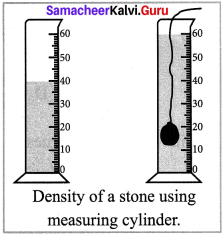1. In order to determine the density of a solid, we must know the mass and volume of the stone.
2. The mass of the stone is determined by a physical balance very accurately. Let it be ‘m’ grams.
3. In order to find the volume, take a measuring cylinder and pour in it some water.
4. Record the volume of water from the graduations marked on measuring cylinder. Let it be 40 cm3.
5. Now tie the given stone to a fine thread and lower it gently in the measuring cylinder, such that it is completely immersed in water.
6. Record the new level of water. Let it be 60 cm3

∴Volume of the solid = (60-40) cm3
= 20 cm3 = V cm3 (assume)
Knowing the mass and the volume of the stone, the density can be calculate by the formula: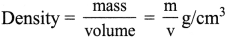XI. Questions based on Higher er Thinking skills:

Question 1.
There are three spheres A, B, C as shown below :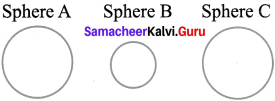Sphere A and B are made of the same material. Sphere C is made of a different material. Spheres A and C have equal radii. The radius of sphere B is half that of A. Density of A is double that of C.

Question 2.

1. Find the ratio of masses of spheres A and B.
2. Find the ratio of volumes of spheres A and B.
3. Find the ratio of masses of spheres A and C.

i. Ratio of masses of spheres A and B
MA : MB
D × VA : D × VB
Let the mass of sphere A = MA
Let the mass of sphere B = MB
Mass = Density × Volume
MA = DA × VA
MB = DB × VB (Density is same)
Volume of Sphere A =$$\frac { 4 }{ 3 }$$πr3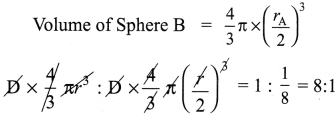ii. Ratio of volumes of spheres A and B
VA : VB
8 : 1 (As mass is directly proportional to volume)

iii. Ratio of masses of spheres A and C.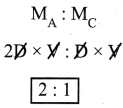[∴ Density of A is double that of C ]

XII. Numerical problems:

Question 1.
A circular disc has a radius 10 cm. Find the area of the disc in m2. (Use n = 3.14)
Given radius = 10 cm = 0.1m
π= 3.14
Area of a circular disc A = ?
Formula : Area of a circle A = πr2
= 3.14 × 0.1 × 0.1
Solution : A = 0.0314 m2

Question 2.
The dimension of a school playground is 800 m x 500 m. Find the area of the ground.
Given :The dimension of a school
Playground = l x b = 800 m x 500 m

Formula :Area of the ground A = l x b
= 800 x 500 = 4,00,000
A = 4,00,000 m2

Question 3.
Two spheres of same size are made from copper and iron respectively. Find the ratio between their masses. Density of copper 8,900 kg/m and iron 7,800 kg/m3
Given : Density Copper Dc = 8900 kg/m3
Density of Iron D1 = 7800 kg/m3
Volume of Copper sphere = Volume of Iron sphere
To find : Ratio of Masses of Copper (MC) and Iron (MI)
Solution: Mass = Density x Volume
MC = DC x V, M1 = D1 x V
MC = 8900 V, M1 = 7,800 V
MC = M1
8900 V : 7800 V
= 1.14: 1

Question 4.
A liquid having a mass of 250 g fills a space of lOOOcc. Find the density of the liquid.
Given : Mass of a liquid M = 250 g
Volume V = l000cc
Density of the liquid D = ?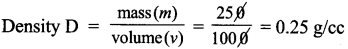Solution: Density of the liquid = 0.25 g/cc

Question 5.
A sphere of radius 1cm is made from silver. If the mass of the sphere is 33 g, find the density of silver (Take π = 3.14)
Given : radius of a sphere r = 1cm
Volume of the sphere V = ?
Mass of the sphere M = 33 g
Density of silver D = ?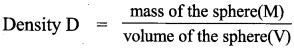Solution: Density of silver sphere = 7.889 g/cc.

XIII. Cross word puzzle:

Clues – Across
1. SI unit of temperature
2. A derived quantity
3. Mass per unit volume
4. Maximum volume of liquid a container can hold

Clues – Down
a. A derived quantity
b. SI unit of volume
c. A liquid denser than iron
d. A unit of length used to measure very long distances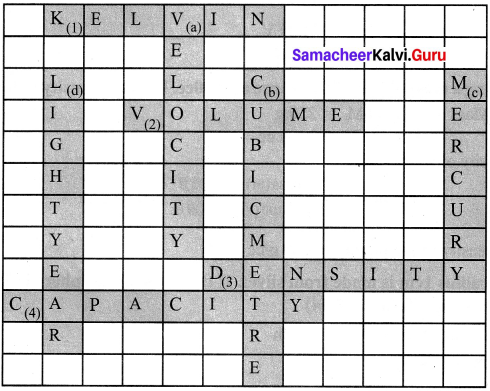Clues – Across
1. KELVIN
2. VOLUME
3. DENSITY
4. CAPACITY

Clues – Down
a. VELOCITY
b. CUBIC METRE
c. MERCURY
d. LIGHT YEAR

### Samacheer Kalvi 7th Science Measurement lntext Activities

Activity -1

Take a leaf from any one of trees in your neighborhood.
Place the leaf on a graph sheet and draw the outline of the leaf with a pencil. Remove the leaf. You can see the outline of the leaf on the graph sheet.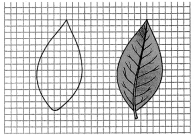1. Now, count the number of whole squares enclosed within the outline of the leaf. Take it to be M.
2. Then, count the number of squares that are more than half. Take it as N.
3. Next, count the number of squares which are half of a whole square. Note it to be P.
4. Finally, count the number of squares that are less than half. Let it be Q.
5. M = _______;N = _______; P = _______; Q = _______

Now, the approximate area of the leaf can be calculated using the following formula:
Approximate area of the leaf = M +($$\frac { 3 }{ 4 }$$) N+($$\frac { 1 }{ 2 }$$) P+($$\frac { 1 }{ 4 }$$) Q square cm
Area of the leaf =________.
This formula can be used to calculate the area of any irregularly shaped plane figures.
M = 50
N = 7
P = 4
Q = 4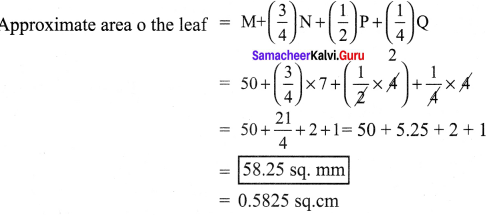Activity – 2

Draw the following regularly shaped figures on a graph sheet and find their area by the graphical method. Also, find their area using appropriate formula. Compare the results obtained in two methods by tabulating them.
(a) A rectangle whose length is 12 cm and breadth is 4 cm.
(b) A square whose side is 6 cm.
(c) A circle whose radius is 7 cm.
(d) A triangle whose base is 6 cm and height is 8 cm.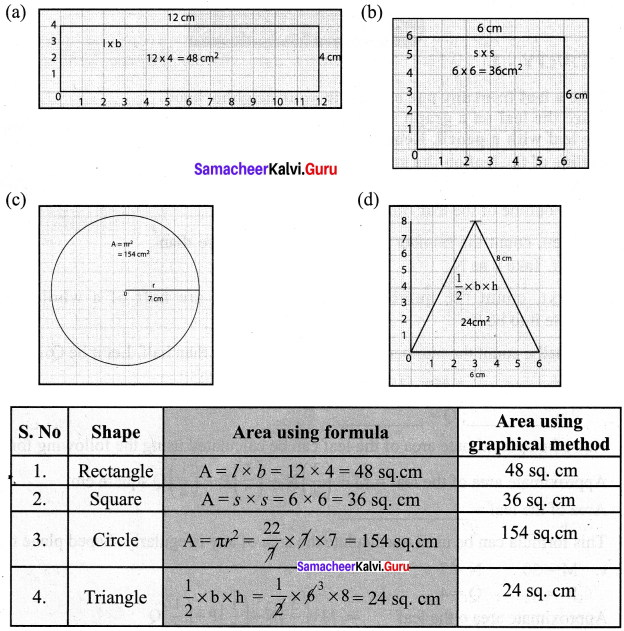Activity – 3

Take a measuring cylinder and pour some water into it (Do not fill the cylinder completely). Note down the volume of water from the readings of the measuring cylinder. Take it as V . Now take a small stone and tie it with a thread. Immerse the stone inside the water by holding the thread. This has to be done such that the stone does not touch the walls of the measuring cylinder. Now, the level of water has raised. Note down the volume of water and take it to be V . The volume of the stone is equal to the raise in the volume of water.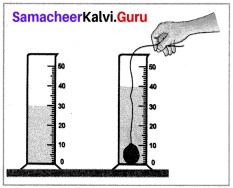V1= _______
V2=_______
Volume of stone = v2 – v1 =_______.
V1 = 30 cc, V2 = 40 cc; Volume of stone =v2 – v1 = 40cc – 30cc = 10cc

Activity – 4
(a) Take an iron block and a wooden block of same mass (say 1 kg each). Measure their volume. Which one of them has more volume and occupies more volume?
(b) Take an iron block and a wooden block of same size. Weigh them and measure their mass. Which one of them has more mass?
(a) Wooden block has more volume and occupies more volume. (As the molecules of wood are loosely packed)
(b) Iron block has more mass. (In iron block, molecules are closely packed).

### Samacheer Kalvi 7th Science Measurement Additional Questions

Question 1.
The unit of volume is _____
(a) m3
(b) m3
(c) cm3
(d) km
(a) m3

Question 2.
Physical quantities are classified into _____ type
(a) three
(b) two
(c) four
(d) none of the above
(b) two

Question 3.
The SI unit of speed is _____
(a) m/s2
(b) m/s
(c) km/h
(d) m2/s
(a) m/s2

Question 4.
1 litre = ______ cc
(a) 100
(b) 1000
(c) 10
(d) 0.1
1000

Question 5.
The formula to calculate area of a rectangle is _____
(b) side × side
(d) none of the above

Question 6.
_____ is a derived quantity.
(a) length
(b) mass
(c) time
(d) area
(d) area

Question 7.
The amount of space occupied by a three dimensional object is known as its _____
(a) density
(b) volume
(c) Area
(d) mass
(b) volume

Question 8.
The maximum volume of liquid that a continer can hold is _____
(a) area
(b) volume
(c) capacity
(d) density
(c) capacity

Question 9.
The shortest distance between the earth and the sun is called as _____ position.
(a) Light year
(b) normal
(c) perihelion
(d) aphelion
(c) Perihelion

Question 10.
The largest distance between the earth and the sun is called as _____ position.
(a) normal
(b) perihelion
(c) aphelion
(d) none of the above
(c) aphelion

II. Fill in the Blanks.

1. The materials with higher density are called ______
2. The materials with lower density are called ______
3. The area of irregularly shaped figures can be calculated with the help of a ______
4. The SI unit of volume is ______
5. The SI unit of density is ______
6. The CGS unit of density is ______
7. If the density of a solid is lower than that of a liquid it ______ is that liquid
8. If the density of a solid is higher than that of a liquid, it ______ is that liquid.
9. The total number of seconds in one year = ______
10. The average distance between the earth and the sun is about ______ million kilometre.

1. denser
2. rarer
3. graph sheet
4. cubic metre or m3
5. kg/m3
6. g/cm3
7. floats
8. sinks
9. 3.15 3 x 107 second
10. 149.6

III. True or False – if false give the correct statement.

Question 1.
One square metre is the area enclosed inside a square of side 2 metre.
(False) Correct Statement: One square metre is the area enclosed inside a square of side 1 metre.

Question 2.
Area is a derived quantity as we obtain by multiplying twice of the fundamental physical quantity length.
True.

Question 3.
Density of water is 100 kg/m3
(False) Correct statement: Density of water is 1000 kg/m3

Question 4.
Density is defined as the mass of the substance contained in unit volume.
True.

Question 5.
The lightness or heaviness of a body is due to volume
(False) Correct statement: The lightness or heaviness of a body is due to density.

Question 6.
Neptune is 30 AU away from sun.
True.

Question 7.
The nearest star to our solar system is proxima centauri.
True.

Question 8.
The volume of a figure is the region covered by the boundary of the figure.
(False) Correct statement: The area of a figure is the region covered by the boundary of the figure.

Question 9.
1 Light year = 9.46 x 105 m.
True.

Question 10.
One light year is defined as the distance travelled by light inW vacuum during the period of one year.
True.

IV. Match the following :
Question 1.

 1 Length (a) ampere (A) 2 time (b) kelvin (K) 3 Mass (c) metre (M) 4 Temperature (d) second (S) 5 Electric current (e) kilogram (K)

1. c
2. d
3. e
4. b
5. a

Question 2.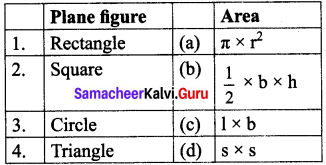1. c
2. d
3. a
4. b

V. Assertion and Reason.

Mark the correct choice as
(a) Both A and R are true but R is not the correct reason.
(b) Both A and R are true and R is the correct reason.
(c) A is true but R is false.
(d) A is false but R is true

Question 1.
Assertion (A): The distance between two celestial bodies is measured by the unit of light year.
Reason (R) : The distance travelled by the light in one year in vacuum is called one light year.
(a) Both A and R are true but R is not the correct reason.

Question 2.
Assertion (A): It is easier to swim in sea water than in river water.
Reason (R) : Density of sea water is more than that of river water
(a) Both A and R are true but R is not the correct reason.
(b) Both A and R are true and R is the correct reason.
(c) A is true but R is false.
(d) A is false but R is true.
(b) Both A and R are true and R is the correct reason.

Question 1.
Write the SI unit of speed.
m/s

Question 2.
What is the fundamental unit of amount of substance?
mole (mol)

Question 3.
What are the types of physical quantity?

1. Fundamental quantity
2. Derived quantity.

Question 4.
What is the SI unit of electric charge?
Coulomb (C)

Question 5.
Mention the formula to calculate area of a circle?
n × r2 = πr2.

Question 6.
How do you find the area of irregularly shaped figures?
Graphical method.

Question 7.
How will you determine the volume of a liquid?
By using measuring cylinder.

Question 8.
What are the other units used to measure the volume of liquids?
Gallon, ounce and quart.

Question 9.
Which one of the following has more volume. Iron block or a wooden block of same mass.
Wooden block.

Question 10.
Which one of the following has more density. Water or cooking oil.
Water

Question 1.
What is fundamental quantity? Give examples.
A set of physical quantities which cannot be expressed in terms of any other quantities are known as fundamental quantities. Ex: Length, mass, time.

Question 2.
Define mass Mention its unit.
Mass is the amount of matter contained in a body. It’s unit is kilogram (kg).

Question 3.
What are the multiples and sub multiples of mass?
The multiples of mass are quintal and metric tonne.
The sub-multiples of mass are gram and milligrams.

Question 4.
What is physical quantity? give example.
A quantity that can be measured is called a physical quantity. For example, the length of a piece of cloth, the time at which school begins.

Question 5.
What do you mean by ‘unit’?
The known measure of a physical quantity is called the unit of measurement.

Question 6.
What is measurement?
Comparison of an unknown quantity with a standard quantity is called measurement.

Question 7.
What is meant by area?
Area is the measure of the region inside a closed line.

Question 8.
What is capacity of a container?
The volume of liquid which a container can hold is called its capacity.

Question 9.
What is the relation between density, volume and mass?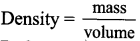Question 10.
Define astronomical unit.
One astronomical unit is defined as the average distance between the earth and the sun. 1AU = 1.496 x 1011 m or 149.6 x 106 m

Question 1.
How will you find the volume of an irregularly shaped object (stone) by using measuring cylinder?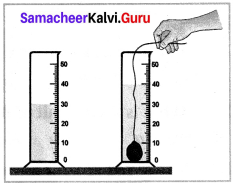1. Take a measuring cylinder and pour some water into it.
2. Note down the volume of water from the readings of the measuring cylinder.
3. Take it as V1
4. Now take Q small stone and tie it with a thread.
5. Immerse the stone inside the water by holding the thread.
6. This has to be done such that the stone does not touch the walls of the measuring cylinder.
7. Now the level of water has raised.
8. Note down the volume of water and take it to be V2

The volume of the stone is equal to the raise in the volume of water.
V1 = 30cc, V2 = 40cc
Volume of stone = V2 – Vj = 40 – 30 = 10cc

IX. Problems for practice:

Question 1.
A piece of iron weighs 230 g and has a volume of 20 cm3. Find the density of iron.
Solution:
Mass of iron (m) = 230 g
Volume of iron (v) = 20 cm3Question 2.
Find the mass of silver of volume 50 cm3 and density 10.5 g / cm3
Solution:
Mass of silver (M) = ?
Volume of silver (V) =50 cm3
Density of silver D = 10.5 g/cm3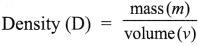mass (M) = Density × Volume
= 10.5 x 50 = 525 g

X. Creative questions: HOTS

Question 1.
Why does an iron needle sink in water, but not an iron ship?